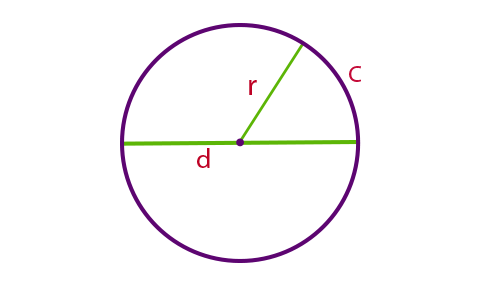# Circumference Formula

The circumference formula of a circle is obtained by multiplying the diameter by pi(π). To recall, the circumference of a closed curve or circular object is the distance around its edge. The circumference of a circle has special importance in geometry and trigonometry.Formula to Calculate Circumference of a Circle

## Formula to Find Circumference

The Circumference Formula requires either the radius or the diameter of the circle for its calculation.

 Circumference of a Circle C = 2πr = πd

Where,

• C is the circumference of the circle.
• d is the diameter of the circle.
• r is the radius of the circle.

### Circumference Formula in Terms of Area

 C = 2√(πA)

### Solved Examples

Question: The radius of a circle is 2 inches. What is the circumference of the circle?

Solution:

Given,

Radius of the circle = r = 2 inches

Circumference of the circle = 2πr = 2 × π × 2 inches = 12.57 inches